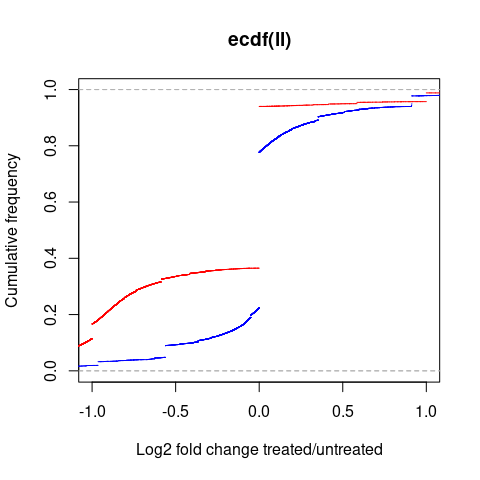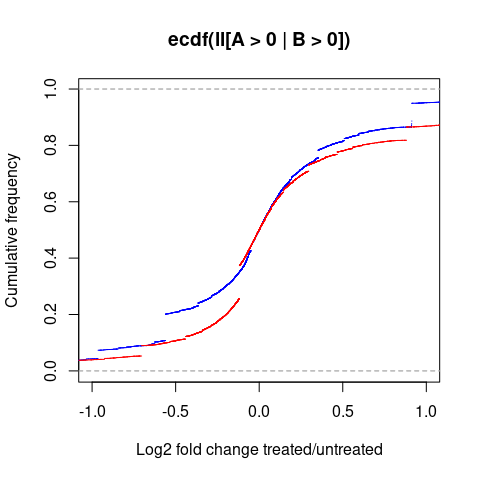# Using LFC

#### 2023-04-19

Digital expression measurements (e.g. RNA-seq) are often used to determine the change of quantities upon some treatment or stimulus. The resulting value of interest is the fold change (often logarithmized).

This effect size of the change is often treated as a value that can be computed as $$lfc(A,B)=\log_2 \frac{A}{B}$$. However, due to the probabilistic nature of the experiments, the effect size rather is a random variable that must be estimated. This fact becomes obvious when considering that $$A$$ or $$B$$ can be 0, even if the true abundance is non-zero.

We have shown that this can be modelled in a Bayesian framework [1,2]. The intuitively computed effect size is the maximum likelihood estimator of a binomial model, where the effect size is not represented as fold change, but as proportion (of note, the log fold change simply is the logit transformed proportion). The Bayesian prior corresponds to pseudocounts frequently used to prevent infinite fold changes by $$A$$ or $$B$$ being zero. Furthermore, the Bayesian framework offers more advanced estimators (e.g. interval estimators or the posterior mean, which is the optimal estimator in terms of squared errors).

This R package offers the implementation to harness the power of this framework.

## Basic usage

The most basic function to estimate effect sizes is

PsiLFC(A,B)

$$A$$ and $$B$$ are vectors of counts, corresponding to the n genes in conditions $$A$$ and $$B$$ (i.e. they are columns from the normal count matrices). What PsiLFC does it to obtain reasonable pseudocounts (see next section), compute the posterior mean for each entry, and then output normalized (i.e. median-centered) effect sizes in the log$$_2$$ fold change representation.

Let’s consider a real world example:

library(DESeq2)
library(lfc)

# it is overly complicated to read a gzipped table from the internet. This is how it is done:

A <- t[,4]
B <- t[,2]

ll <- PsiLFC(A,B)
plot(ecdf(ll),xlim=c(-1,1),xlab="Log2 fold change treated/untreated",ylab="Cumulative frequency",col='blue')
lines(ecdf(CenterMedian(log2((A+1)/(B+1)))),col='red')This shows the log fold change distributions estimated by the posterior mean (blue) and the intuitive way using pseudocounts of 1 (red). This distribution is heavily distorted by several genes with no reads in any of the two conditions. Interestingly, the intuitive way of computing effect sizes results in an asymmetric distribution with more downregulated than upregulated genes.

plot(ecdf(ll[A>0 | B>0]),xlim=c(-1,1),xlab="Log2 fold change treated/untreated",ylab="Cumulative frequency",col='blue')
lines(ecdf(CenterMedian(log2((A+1)/(B+1))[A>0 | B>0])),col='red')Here, only genes are considered that have at least one read in one of the two conditions. The intuitive fold changes still appear to overestimate changes, as well as show more artifacts.

Also, this package provides a drop-in replacement for DESeq2’s results function:

dds <- DESeqDataSetFromMatrix(countData = t[,2:5],colData = data.frame(IFN=c("0h","0h","1h","1h")),design= ~ IFN)
dds <- DESeq(dds)
res <- results(dds, contrast=c("IFN","1h","0h"),cre=TRUE)
head(res)
## DataFrame with 6 rows and 9 columns
##      baseMean log2FoldChange     lfcSE      stat    pvalue      padj     PsiLFC
##     <numeric>      <numeric> <numeric> <numeric> <numeric> <numeric>  <numeric>
## 1  4766.59583     -0.0362689  0.257887 -0.140639  0.888155  0.999982 -0.0995897
## 2     0.00000             NA        NA        NA        NA        NA  0.0000000
## 3   317.39766     -0.1228447  0.339650 -0.361680  0.717591  0.999982 -0.2528005
## 4 65750.14970     -0.0564711  0.360087 -0.156826  0.875382  0.999982 -0.2263070
## 5    30.14576     -0.2019444  0.703263 -0.287153  0.773995  0.999982 -0.3382438
## 6     9.22182      0.4037104  1.127183  0.358159  0.720224  0.999982  0.2437406
##   Credible 0.05 Credible 0.95
##       <numeric>     <numeric>
## 1     -0.134204    -0.0650096
## 2     -1.351969     1.3120596
## 3     -0.386212    -0.1200290
## 4     -0.235576    -0.2170414
## 5     -0.755945     0.0726078
## 6     -0.425486     0.9077995

Important: To make this work, load lfc after DESeq2!

Note that here we also computed the 90% credible interval. The parameter cre can also be given to PsiLFC or PsiLFC.se!